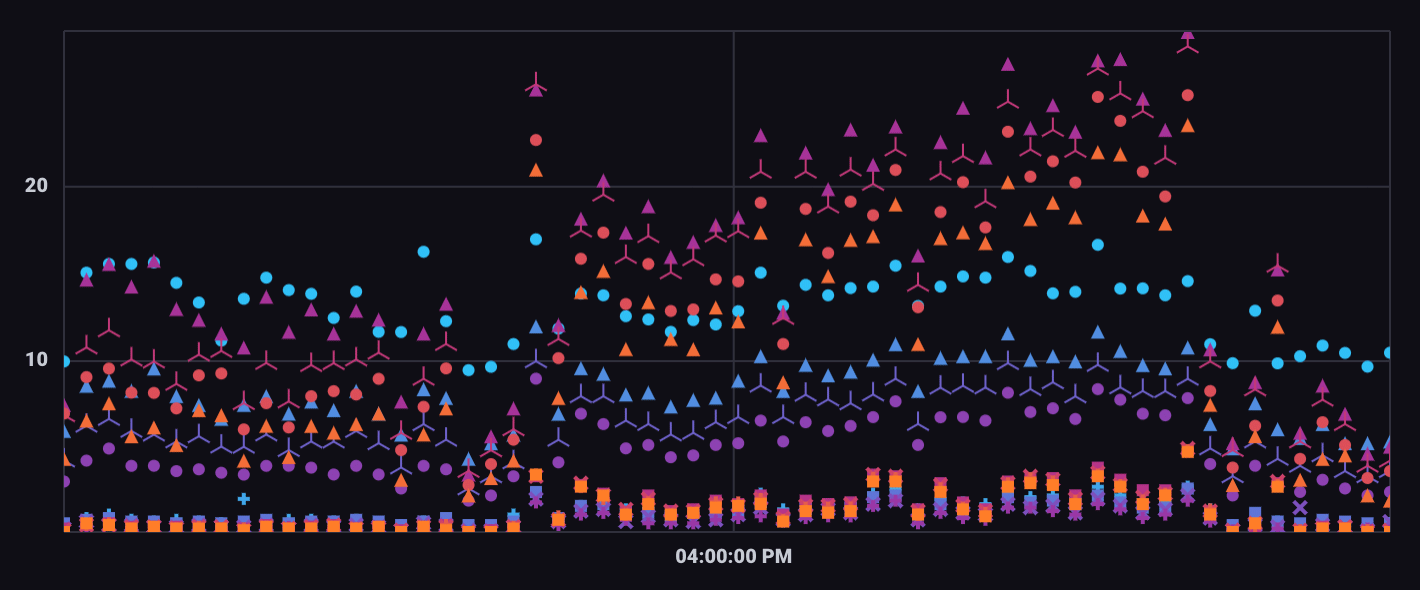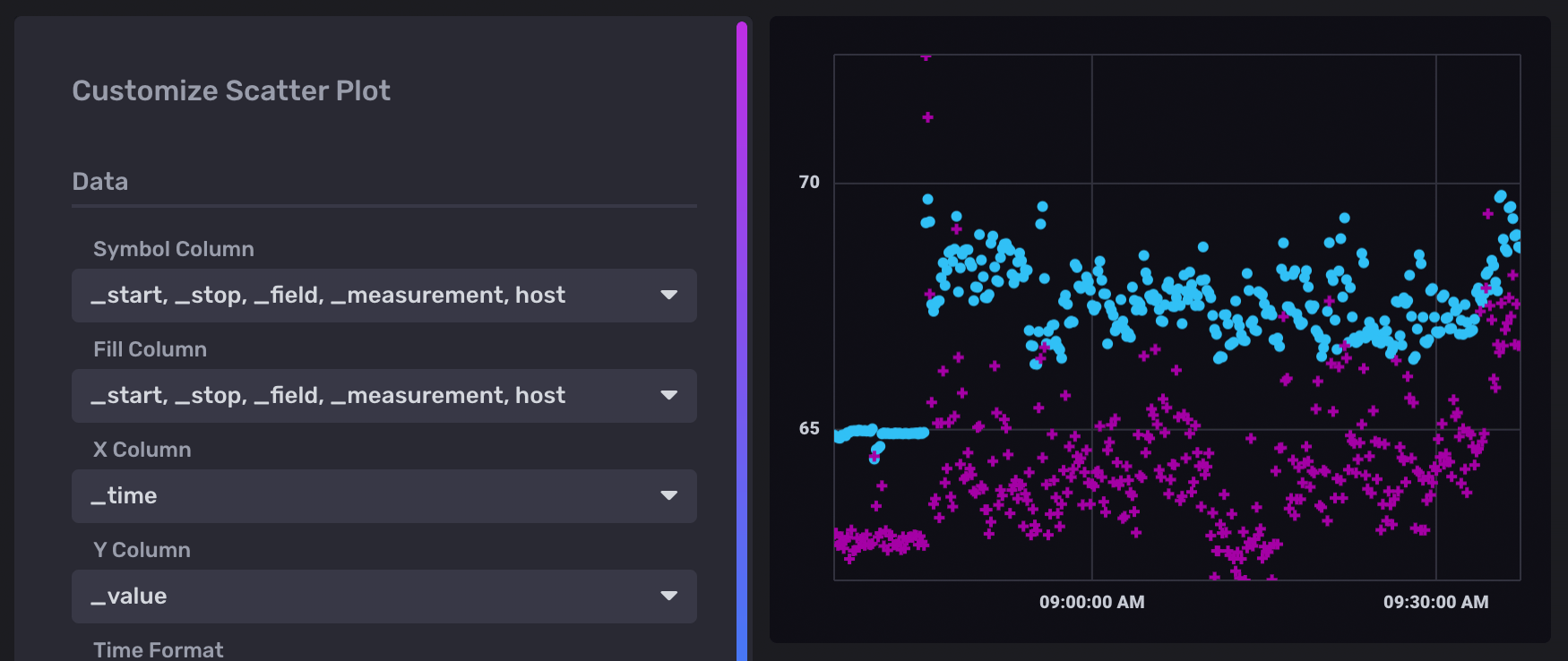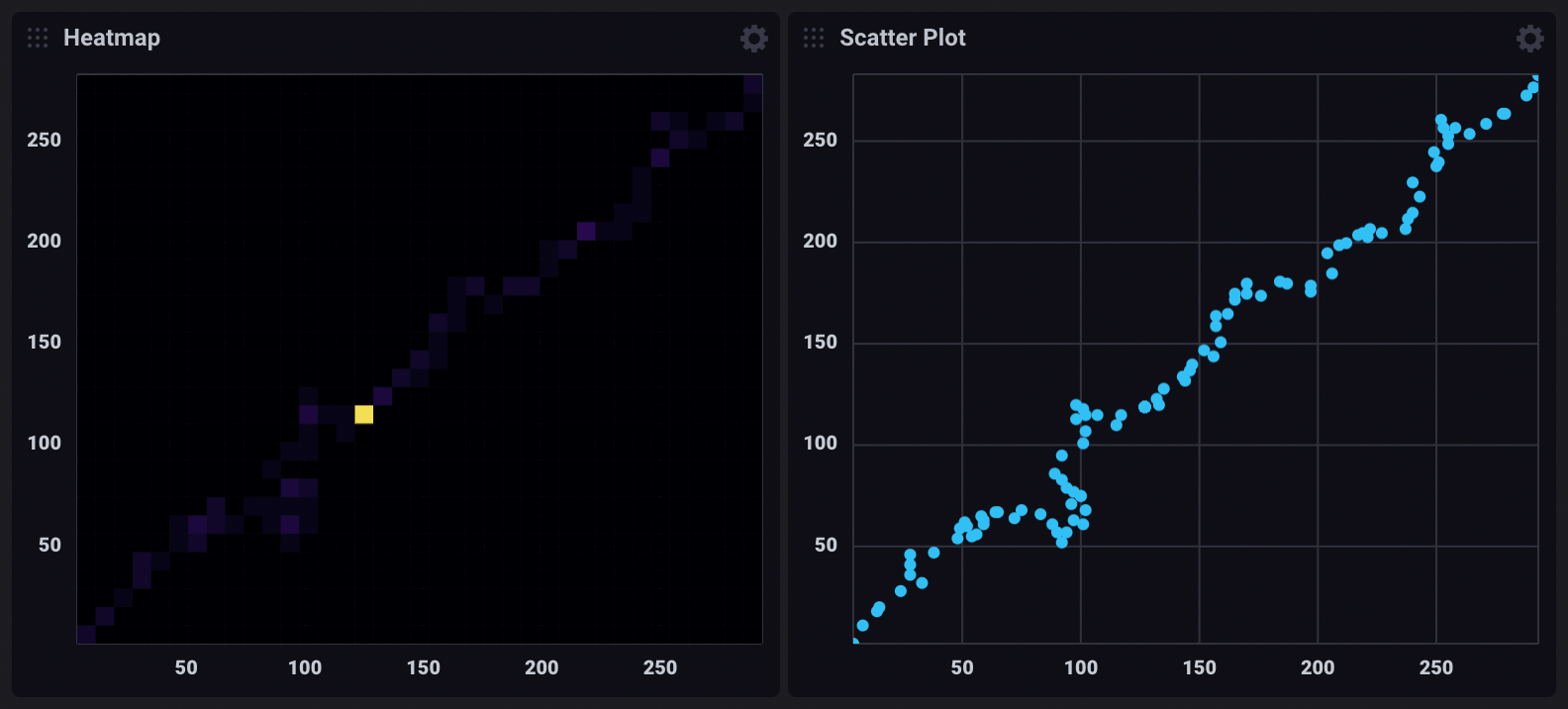# Scatter visualization

The Scatter view uses a scatter plot to display time series data.Select the Scatter option from the visualization dropdown in the upper right.

## Scatter behavior

The scatter visualization maps each data point to X and Y coordinates. X and Y axes are specified with the X Column and Y Column visualization options. Each unique series is differentiated using fill colors and symbols. Use the Symbol Column and Fill Column options to select columns used to differentiate points in the visualization.

## Scatter controls

To view Scatter controls, click Customize next to the visualization dropdown.

###### Data
• Symbol Column: Define a column containing values that should be differentiated with symbols.
• Fill Column: Define a column containing values that should be differentiated with fill color.
• X Column: Select a column to display on the x-axis.
• Y Column: Select a column to display on the y-axis.
###### Options
• Color Scheme: Select a color scheme to use for your scatter plot.
###### X Axis
• X Axis Label: Label for the x-axis.
###### Y Axis
• Y Axis Label: Label for the y-axis.
• Y Tick Prefix: Prefix to be added to y-value.
• Y Tick Suffix: Suffix to be added to y-value.
• Y Axis Domain: The y-axis value range.
• Auto: Automatically determine the value range based on values in the data set.
• Custom: Manually specify the minimum y-axis value, maximum y-axis value, or range by including both.
• Min: Minimum y-axis value.
• Max: Maximum y-axis value.

## Scatter examples

### Cross-measurement correlation

The following example explores possible correlation between CPU and Memory usage. It uses data collected with the Telegraf Mem and CPU input plugins.

###### Query CPU and memory usage

The following query creates a union of CPU and memory usage. It scales the CPU usage metric to better align with baseline memory usage.

``````cpu = from(bucket: "example-bucket")
|> range(start: v.timeRangeStart, stop: v.timeRangeStop)
|> filter(fn: (r) =>
r._measurement == "cpu" and
r._field == "usage_system" and
r.cpu == "cpu-total"
)
// Scale CPU usage
|> map(fn: (r) => ({
_value: r._value + 60.0,
_time: r._time
})
)

mem = from(bucket: "example-bucket")
|> range(start: v.timeRangeStart, stop: v.timeRangeStop)
|> filter(fn: (r) =>
r._measurement == "mem" and
r._field == "used_percent"
)

union(tables: [cpu, mem])
``````
###### Use a scatter plot to visualize correlation

In the Scatter visualization controls, points are differentiated based on their group keys.## Important notes

### Differences between a scatter plot and a heatmap

Scatter plots and Heatmaps both visualize the distribution of data points on X and Y axes. However, in certain cases, scatterplots can “hide” points if they share the same X and Y coordinates.

For example, the dashboard cells below visualize the same query results:The heatmap indicates isolated high point density, which isn’t visible in the scatter plot. In the scatter plot visualization, points that share the same X and Y coordinates appear as a single point.

New! Cloud or OSS?